• 多元统计分析数据集
千次阅读
2020-04-17 18:51:25

xbar=colMeans(x)#样本均值向量#
y=cov(x)#样本协方差#
m=(n-1)y#li离差#
n=cor(x)#相关矩阵#
u=c(7,5,4,8)
T2=19
t(xbar-u)%%solve(n)%%(xbar-u)#统计量#
16/(19*4)*T2#F#
qf(1-0.05,4,16)#F分布临界值0.05#

更多相关内容
• applied multivariate statistical analysis 里用到的数据
• 何晓群 多元统计分析 第四版数据 有典型相关分析 因子分析 主成分分析等
• R语言案例分析：多元数据的基本统计分析数据集下载 我们利用该数据集中的Case1来完成下面的R语言操作： > options(digits = 4) #输出结果位数> par(mar=c(4, 4, 2, 1) + 0.1, cex=0.8) # 图形修饰> case1 > head...

R语言案例分析：多元数据的基本统计分析

来自《多元统计分析与R语言建模》 第四版

数据集下载

我们利用该数据集中的Case1来完成下面的R语言操作：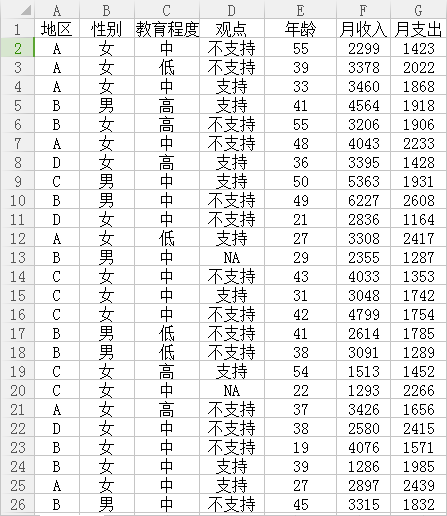options(digits = 4) #输出结果位数
par(mar=c(4, 4, 2, 1) + 0.1, cex=0.8) # 图形修饰
head(case1)
地区 性别 教育程度    观点     年龄 月收入 月支出
1    A      女       中            不支持   55   2299   1423
2    A      女       低   不支持   39   3378   2022
3    A      女       中   支持       33   3460   1868
4    B      男       高   支持       41   4564   1918
5    B      女       高  不支持    55   3206   1906
6    A      女       中  不支持    48   4043   2233

summary(case1)
地区    性别     教育程度     观点                           年龄              月收入               月支出

A:204   男:603   低:319   不支持:628       Min.   : 6.0          Min.   : 637         Min.   : 797

B:401   女:597   高:303   支持  :568   1st Qu.:34.0      1st Qu.:2388      1st Qu.:1722

C:384                 中:578    NA's  : 4        Median :40.0    Median :2978   Median :1993

D:211                         Mean   :40.1      Mean   :3006     Mean   :1997

3rd Qu.:47.0      3rd Qu.:3624     3rd Qu.:2262

Max.   :72.0        Max.   :6239      Max.   :3385

# 定性分析
attach(case1) #绑定数据T1 <- table(地区)

T1

A    B    C    D
204 401 384 211
barplot(T1) #绘制条形图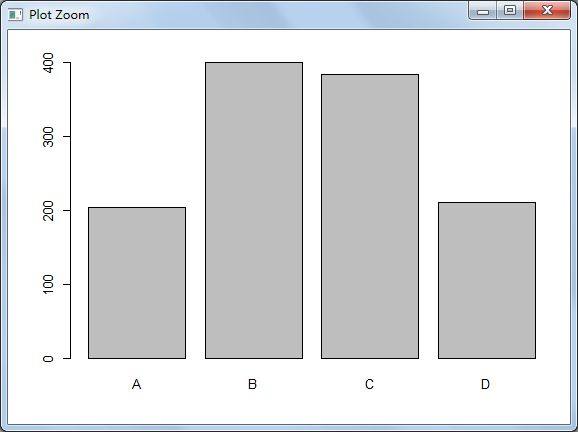# 定量分析
f <- hist(月收入) #直方图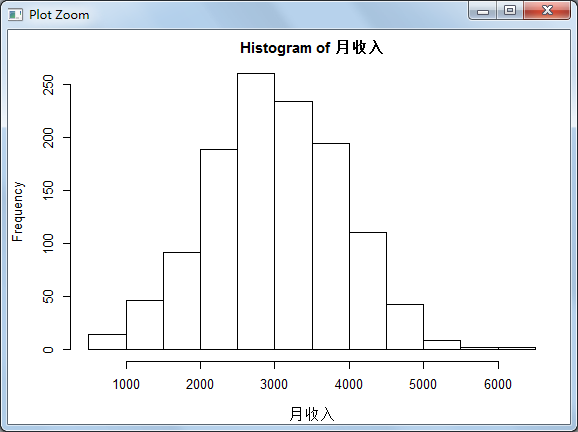# 定性定量分析
boxplot(月收入~性别) #箱线图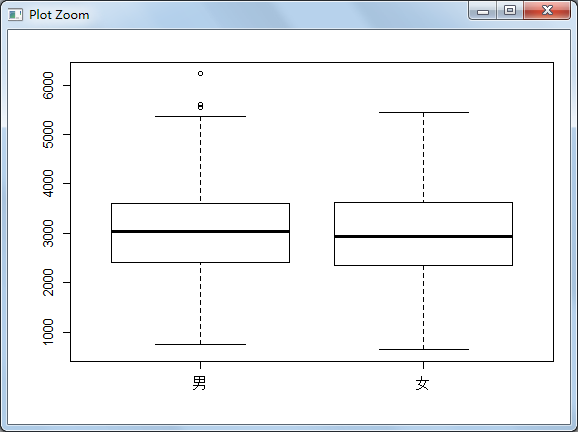t.test(月收入~性别) #t检验Welch Two Sample t-test
data:  月收入 by 性别
t = 0.51, df = 1200, p-value = 0.6
alternative hypothesis: true difference in means is not equal to 0
95 percent confidence interval:
-75.43 128.49
sample estimates:
mean in group 男 mean in group 女
3019                      2993

# 接受男女的月收入无显著差异的假设（p>0.5）

# 二维列联表分析
T2 <- table(性别, 观点)
T2        观点
性别 不支持 支持
男    319    282
女    309    286

barplot(T2, beside = TRUE) # 条形图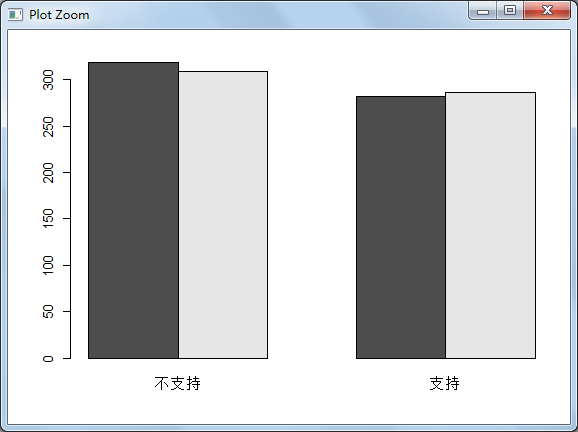barplot(T2, beside = F) # 条形图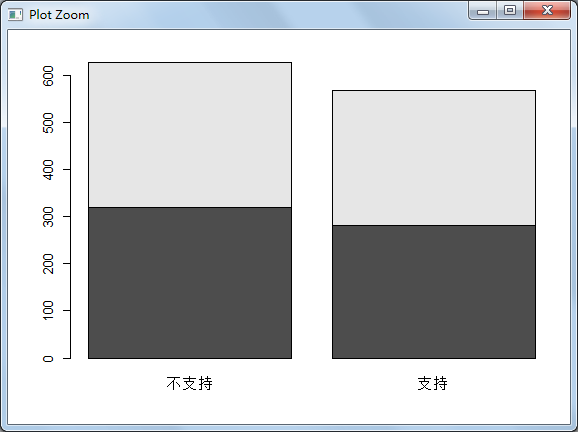# beside=T表示绘制分组条形图，beside=F表示绘制堆叠条形图

# 多维列联表分析
T3 <- ftable(性别, 教育程度, 观点) # 创建一个紧凑的"平铺"式列联表
T3                      观点     不支持 支持
性别    教育程度
男              低                81     88
高                78     66
中                160  128
女     低                82     68
高                86     72
中                141  146

barplot(T3, beside = TRUE, col = 3:4) #条形图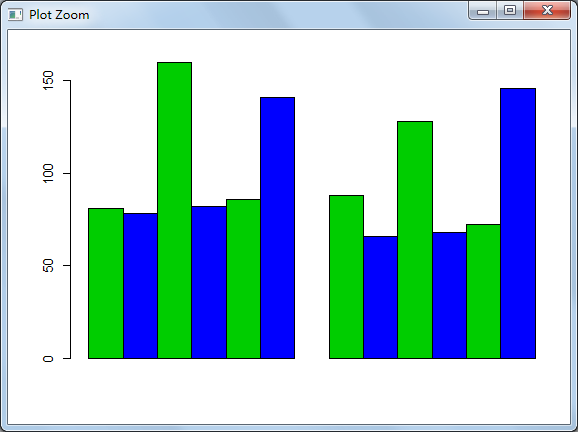T4 <- ftable(教育程度, 性别, 观点)
T4                           观点 不支持  支持
教育程度      性别
低       男           81     88
女           82     68
高       男           78     66
女           86     72
中       男           160  128
女           141  146

barplot(T4, beside = TRUE, col = 3:4) #条形图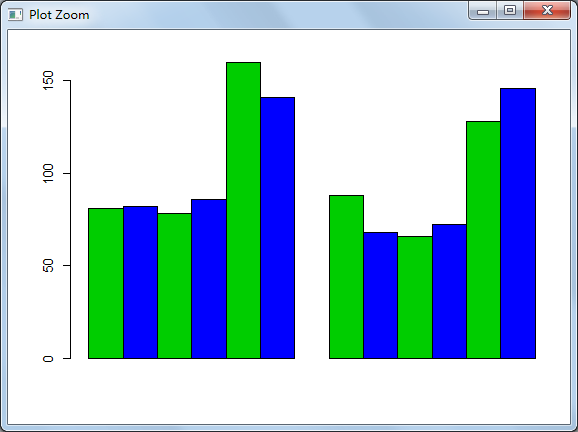detach(case1) #解除绑定



展开全文R语言
• 1.多元数据的数学表示 2.多元数据的直观分析：通过图示的方法显示数据，即是多元数据的可视化。...6.判别分析：传统的多元统计分析方法。对所研究的对象进行判别分类。要求事先归类，对新的对象判断是哪一类……

# 多元统计分析及R语言建模

## 引言：

1.真正结合数据和创造力，需要掌握数据分析与统计建模工具。
理论+实践；
定性+定量；
资料获取：Rstat.leanote.com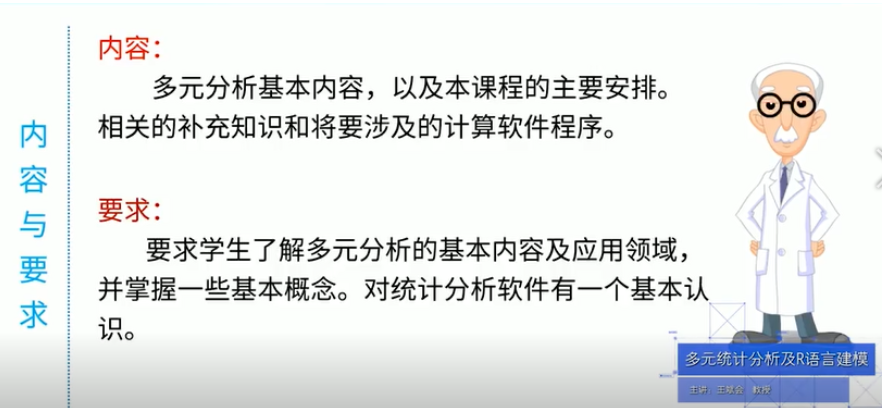## 第1章 多元统计分析的概述

### 1.1 多元统计分析简介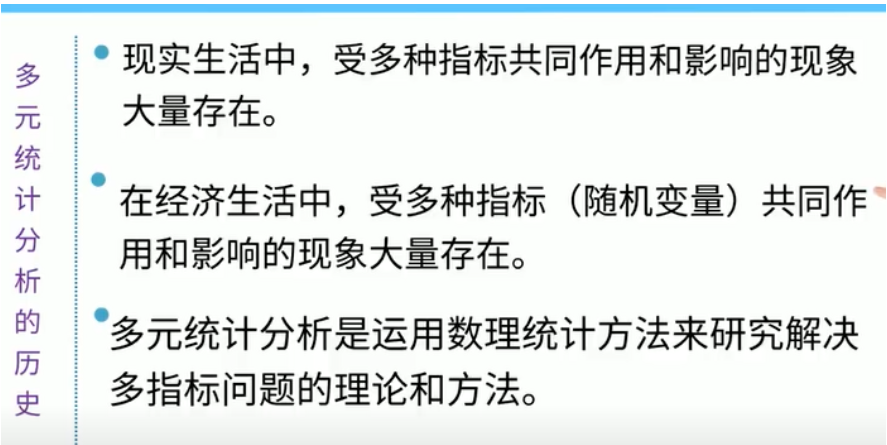### 1.2 多元统计分析用途

1.变量之间的相依性的分析： 研究变量之间的相互关系
2.构造预测模型，进行预报控制： 比如构造回归模型来进行预测，进一步进行估计，控制。
3.进行数值分类，构造分类模式
4.简化系统结构，探讨系统内核

😁重点在于：如何选择适合的方法来解决实际问题，需要对问题进行综合考虑。

### 1.3 多元统计分析内容

1.多元数据的数学表示
2.多元数据的直观分析：通过图示的方法显示数据，即是多元数据的可视化。
3.多元线性相关分析：研究数据之间有没有线性关系
4.多元线性回归分析：在多元线性相关分析基础上，数据之间有相关关系，就用回归分析表示出来。
5.广义和一般线性模型：对多元线性回归分析模型的推广，分成广义和一般线性模型。
6.判别分析：传统的多元统计分析方法。对所研究的对象进行判别分类。要求事先归类，对新的对象判断是哪一类。
7.聚类分析：事先不需要归类，对多变量（多元）的数据进行统计分析。比如计算我们国家31个地区的居民消费，经济发展，就用聚类分析方法研究多变量字节的分类问题。
8.主成分分析：数学技术，变量变化，压缩。把多变量数据（多维的）压缩成一维的，平面上展示。
9.因子分析:主成分分析的推广，给压缩后的数据经济学上或者统计学上的实际意义，表示主成分代表什么。
10.对应分析：因子分析的推广，把R型因子分析，和Q型因子分析，即把样品的因子分析和变量的因子分析合成一个来研究样品和变量之间的关系。
11.典型相关分析：多元线性相关分析的推广。自变量和因变量都是多组的。
12.多维标度法
13.综合评价法

### 1.4 统计软件及其应用

😁强大的统计分析软件

1. SAS: 三大著名分析软件之一，
组合比较困难，
入门比较困难

2. SPSS: 操作简单
无需编程
有翻边的数据接口
灵活的功能模块组合

3. 3 .S-PLUS：操作界面简单
全面的统计模型和分析手段
很强的图形处理能力
兼容性极好

🙄完整的数值计算软件

MATLAB:

• ​ 数值分析
• ​ 数值和符号计算
• ​ 工程与科学绘图
• ​ 控制系统的设计与模仿
• ​ 数字图像处理技术
• ​ 数字信号处理技术
• ​ 通讯系统设计与仿真
• ​ 财务与金融工程

### 1.5 R语言及系统设置

😁免费的数据分析软件

R:

• 功能强大
• 免费，开源
• 前景广阔

1.优点：自由软件，统计功能强大，可以看做是Splus的免费版本，是统计研究的首选
2.缺点：初学较为麻烦，需要有一一定的编程经验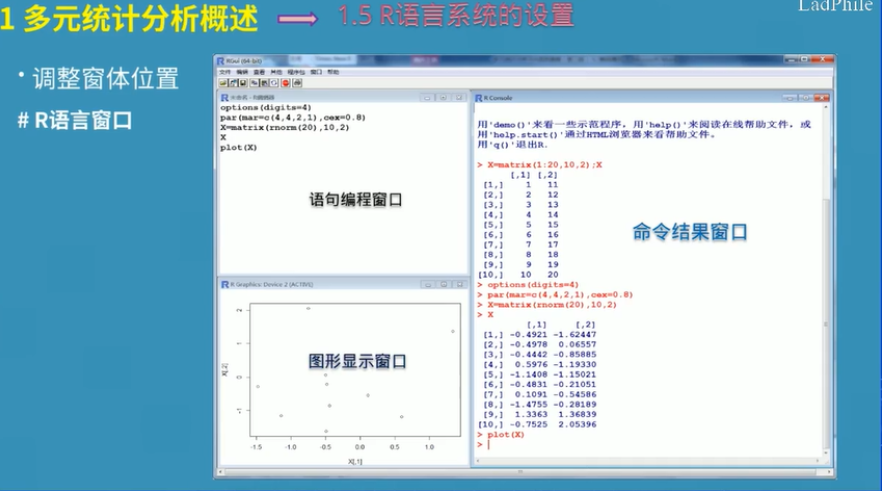• 在语句编程窗口Ctrl+R就可以执行命令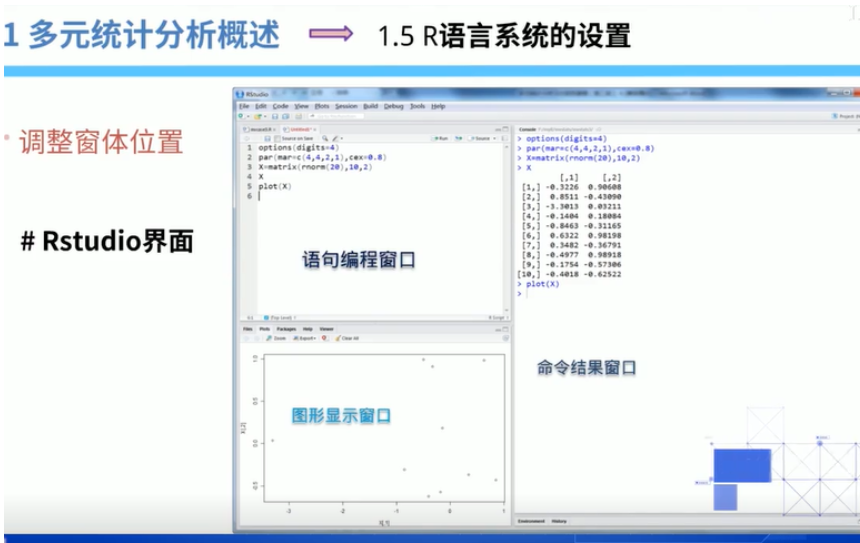### 1.6 R语言包及其使用

01 关于R语言1.R语言是一种为统计计算和图形显示而设计的语言环境，是贝尔实验室开发的S语言的一种实现，可以看成S++，提供了一系列统计的图形显示工具

2.R预言师属于CNU系统的一个自由，免费，源代码开放的软件，是一个用于统计计算和统计制图的1优秀工具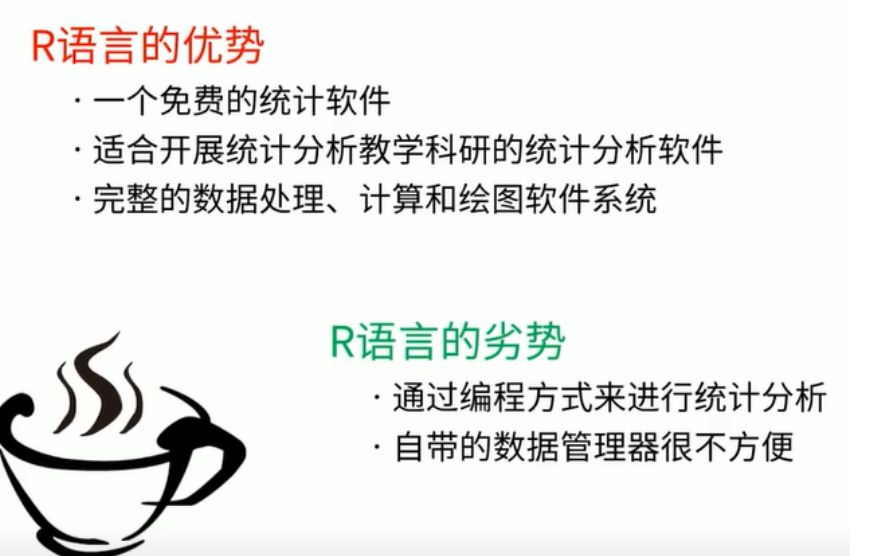#### 02 R语言软件的下载与安装#### 03 R语言包及其函数

R语言标准包：标准（基本）包构成R源代码的一个重要部分。他们包括允许R工作的基本函数和数据集，标准统计和图形工具。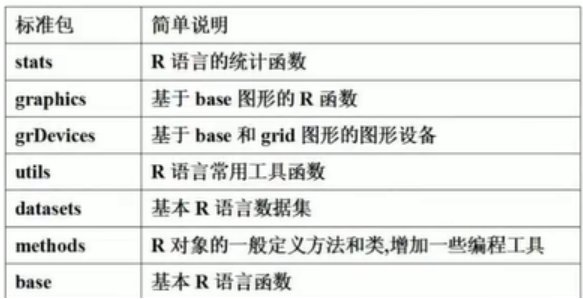#### 04 R语言扩展包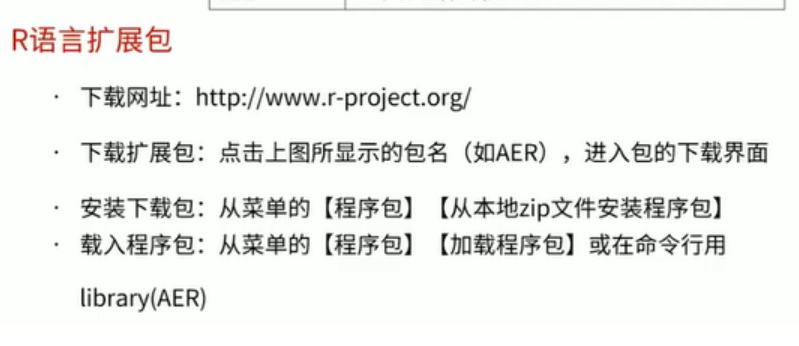展开全文r语言 回归 开发语言
• 1. 经典的线性回归分析与交叉验证 examDict={' 学习时 ':[0.50, 0.75, 1.00, 1.25,1.50,1.75, 1.75,2.00, 2.25,2.50, 2.75,3.00,3.25,3.50,4.00,4.25,4.50,4.75,5.00,5.50], '分':[10,22,13 ,43,20,22,33,50,62 ,...
1. 经典的线性回归分析与交叉验证
examDict={' 学习时 ':[0.50, 0.75, 1.00, 1.25,1.50,1.75, 1.75,2.00, 2.25,2.50,
2.75,3.00,3.25,3.50,4.00,4.25,4.50,4.75,5.00,5.50], '分':[10,22,13 ,43,20,22,33,50,62 ,
48,55,75,62,73,81,76,64,82,90,93]}
examDf = pd.DataFrame(examDict)
X_train,X_test,Y_train,Y_test = train_test_split(exam_X,exam_Y,train_size=0.8)
model = LinearRegression()
model.fit(X_train,Y_train)
a = model.intercept_#截距
b = model.coef_#回归系数
y_train_pred = model.predict(X_train) #预测
score = model.score(X_test,Y_test) #可决系数 0.8866470295386657
2. 经典的多元线性回归分析的模型参数的假设检验
import statsmodels.api as sm
from sklearn import datasets ## 从 scikit-learn 导入数据集
import numpy as np
import pandas as pd
df = pd.DataFrame(data.data, columns=data.feature_names)
target = pd.DataFrame(data.target, columns=["MEDV"])
X = df[['CRIM', 'ZN', 'INDUS']] ## X 通常表示我们的输入变量 (或自变量)
y = target["MEDV"] ## Y 通常表示输出/因变量
model = sm.OLS(y, X).fit() ## sm.OLS(输出, 输入)
predictions = model.predict(X)
model.summary() ## 打印出统计模型

3. 岭回归模型

X_train,X_test,Y_train,Y_test = train_test_split(df2,df1,train_size=0.8)
model = Ridge(alpha=0.5,fit_intercept=True)
model = RidgeCV(alphas=[0.01,0.1,0.2, 0.5, 1],normalize = True,cv=10)
model.fit(X_train,Y_train)
ridge_best_alpha = model.alpha_ #得到最佳lambda值
print(f"岭回归关键正则参数={ridge_best_alpha}")
计算可决系数
a=model.intercept_
b=model.coef_
y_train_pred =model.predict(X_train)
score=model.score(X_test, Y_test)
print(score)

4. 基于最佳lambda值建模

ridge = Ridge(alpha = ridge_best_alpha,normalize = True)
ridge.fit(X_train,Y_train)
ridge_predict = ridge.predict(X_test)
计算损失函数
rmse = np.sqrt(mean_squared_error(Y_test,ridge_predict))

5.  LASSO回归模型：

lasso_cv = LassoCV(alphas = alphas, normalize=True, cv = 10, max_iter=10000)
lasso_cv.fit(x_tr,y_tr)
lasso_best_alpha = lasso_cv.alpha_
lasso_best_alpha
lasso = Lasso(alpha = lasso_best_alpha, normalize=True, max_iter=10000)
lasso.fit(x_tr, y_tr)
lasso_predict = lasso.predict(x_te) #预测
RMSE = np.sqrt(mean_squared_error(y_te,lasso_predict))

本次任务额外知识点：

seed = 7
np.random.seed(seed)
10折交叉验证
kfold = StratifiedKFold(n_splits=10, shuffle=False, random_state=seed)
固定random_state后，每次构建的模型是相同的、生成的数据集是相同的、每次的拆分结果也是相同的

y代表输出答案，y_代表标准答案
mse=tf.reduce_mean(tf.square(Y_test-yy_train_pred))

题目

3. 数据集简介
原始数据有 14 个变量的 506 个观察值，其中， medv( 自住房屋房 价中位数，单位: 千美元 ) 是原始的目标变量，其他变量包括 :crim( 城镇 的人均犯罪率) mn( 占地面积超过 25000 平方英尺的住宅用地的比例 )、 indus(每个镇的非零售业务比例，单位 : 英亩 ) chas( 有关查尔斯河的 虚拟变量，如果挨着河为1 ，否则为 0) mo( 一氧化氮浓度，单位 :Ppm) 、 m(平均每间住房的房间数量 )、 age(1940 年以前建成的自住单位的房 龄比例) dis( 五个波土顿就业中心的加权距离 ) rad( 高速公路的可达 性指数) tax( 每万美元全价物业值的财产税率 ) ptratio( 城镇学生与教 师的比例) b(=100078-0.63)2 ，其中的 B 是城镇黑人的比例 ) Istat( 低 收入人口比例); 更正过的数据集有以下附加变量 :cmed( 修正了的自住 房价中位数，单位: 千美元 ) tow( 镇名称 ) trat( 人口普查区 ) lon(
口普查区的经度 ) lat( 人口普查区的纬度 )
4. 数据集使用
我们将用 comedy ( 修正了的自住房屋房价中位数 ) 作为 因变量 ， 而将 crim zn indus nox rm age dis rad tax ptratio b lstat 12 个变量作为 自变量 。(数据详见 BostonHousing2.csv 文件 )
5. 回归任务指定
（1）利用指定的 12 个自变量与因变量 comedy 创建散布图矩阵， 要目的查看各自变量与因变量之间的相关性
（2）随机地将当前数据集按照 3:1 的容量比例划分为训练集（用于建立模型）和测试集( 用于检测模型的 预测精度 ) ，重复此步骤十次， 并将得到十次结果制作如下的折线图，其中横坐标为次数，纵坐标为 对应次数的可决系数。如下图所示（ 可以与图不一致，主要体现可决 系数变化规律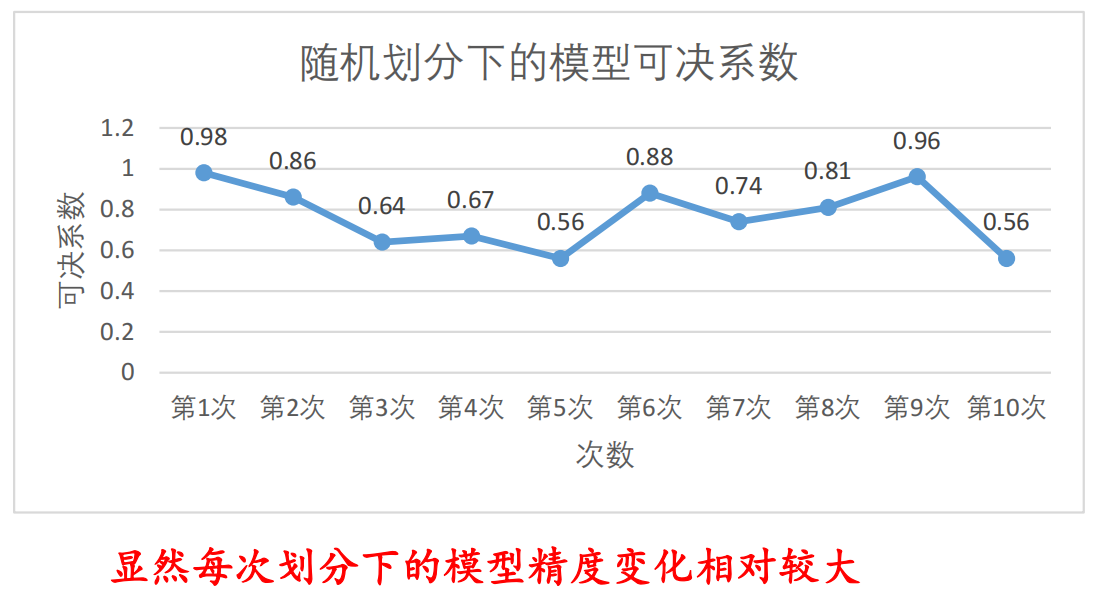（3） 最优回归方程的选择 ：从 12 个自变量中随机的抽取 n （其中 n =2,…..12 ）个自变量，并利用十折交叉验证计算所建模型的可决系 数，依据以上 12 个模型的可决系数大小确定哪一个模型的预测精度 较高。（ 并不一定使用全部自变量的模型精度最好
（4）岭回归、 Lasso 回归模型中关键正则参数 𝛌 的选择：在给定参数 𝛌 的 0.01 0.1 0.2, 0.5, 1 这五个可能性取值的条件下，利用十折交 叉验证和可决系数确定两个模型的各自最优参数 𝛌
（5）在最优参数 𝛌 的条件下的 Lasso 回归模型、岭回归及使用全部 12 个自变量模型的 可决系数 十折交叉验证得到的 ）的对比，在此数据 集上哪一个模型的精度最高呢？在取定最优参数 𝛌 的条件下 Lasso 回归模型中，计算 回归系数为零的个数与全部自变量个数（即，12）的比例。
全部代码
import numpy as np
import random
# import matplotlib.pyplot as plt
import matplotlib
import pandas as pd
from pandas.plotting import scatter_matrix
from sklearn.linear_model import Ridge,RidgeCV
from sklearn.linear_model import LinearRegression
from sklearn.model_selection import train_test_split
from matplotlib import pyplot as plt #as就是重新命名的意思
from matplotlib.font_manager import FontProperties #导入中文字体
from sklearn.linear_model import Lasso,LassoCV
from sklearn.metrics import mean_squared_error
import numpy as np
from sklearn.model_selection import StratifiedKFold

#font = FontProperties(fname=r"/usr/share/fonts/opentype/noto/NotoSansCJK-Regular.ttc")
matplotlib.rcParams['font.family']='SimHei'
matplotlib.rcParams['font.sans-serif']=['SimHei']

#print(pd_data)

df1=pd_data[['cmedv']]
df2=pd_data[['crim', 'zn', 'indus',
'nox', 'rm', 'age', 'dis', 'rad', 'tax', 'ptratio', 'b',
'lstat']]

# #任务一:
print("***************************************************************")
print("任务一")

dff=pd_data[['crim', 'zn', 'indus',
'nox', 'rm', 'age', 'dis', 'rad', 'tax', 'ptratio', 'b',
'lstat','cmedv']]
plt.figure() #自变量
scatter_matrix(dff, alpha = 0.3, figsize = (14,8));
plt.grid(True)
plt.savefig('cmedy')
plt.show()

##任务二:
print("***************************************************************")
print("任务二")

li=[]
for epoch in range(10):
X_train,X_test,Y_train,Y_test = train_test_split(df2,df1,train_size=0.8)

model = Ridge(alpha=0.5,fit_intercept=True)
model = RidgeCV(alphas=[0.1, 1.0, 10.0]) # 通过 RidgeCV 使用交叉验证获取最佳参数值
model.fit(X_train,Y_train)

a=model.intercept_
b=model.coef_
y_train_pred =model.predict(X_train)
score=model.score(X_test, Y_test)
li.append(score)
x = list(range(1, 11, 1))
y = [round(i,2) for i in li]

plt.plot(x, y,  linewidth=2, color='g', marker='o',
markerfacecolor='blue', markersize=3)

#绘制图片
plt.plot(x,y)
plt.ylim(0, 1) # 限定纵轴的范围

for a, b in zip(x, y):
plt.text(a, b, b, ha='center', va='bottom', fontsize=20)
plt.title("房价预测")
plt.xlabel("训练轮数")
plt.ylabel("可决系数")
plt.show()

#任务三  (包含任务5)

#利用岭回归模型随机选取变量进行十折交叉，计算可决系数
print("任务三  (包含任务5)")
print("下面对变量进行随机抽取：")
X_train,X_test,Y_train,Y_test = train_test_split(df2,df1,train_size=0.8)
for p in range(10):
ans=random.randint(1, 12)

df3 = X_train.sample(n=ans,axis=1)
l=list(df3.columns)
df4=pd.DataFrame(X_test,columns=l)

model = Ridge(alpha=0.5,fit_intercept=True)
model = RidgeCV(alphas=[0.01,0.1,0.2, 0.5, 1],normalize = True,cv=10)
model.fit(df3,Y_train)
ridge_best_alpha = model.alpha_ #得到最佳lambda值

a=model.intercept_
b=model.coef_
yy_train_pred =model.predict(df3)

score = model.score(df4,Y_test)
print(f"第{p+1}轮,随机抽取{ans}个变量\n岭回归关键正则参数={ridge_best_alpha},可决系数{round(score,2)}")

#任务四   (包含任务5)
print("*************************************************")
print("任务四  (包含任务5)")
print(f"岭回归回归模型中关键正则参数𝛌的选择:")
X_train,X_test,Y_train,Y_test = train_test_split(df2,df1,train_size=0.8)
model = Ridge(alpha=0.5,fit_intercept=True)
model = RidgeCV(alphas=[0.01,0.1,0.2, 0.5, 1],normalize = True,cv=10)
model.fit(X_train,Y_train)
ridge_best_alpha = model.alpha_ #得到最佳lambda值
print(f"岭回归关键正则参数𝛌={ridge_best_alpha}")

ridge = Ridge(alpha = ridge_best_alpha,normalize = True)
ridge.fit(X_train,Y_train)
ridge_predict = ridge.predict(X_test)
mse = np.sqrt(mean_squared_error(Y_test,ridge_predict))
score=model.score(X_test, Y_test)
print(f"在最优参数𝛌的条件下,损失值{round(mse,2)},可决系数:{round(score,2)}")

print("*************************************************")
print(f"LASSO回归模型中关键正则参数𝛌的选择:")
X_train,X_test,Y_train,Y_test = train_test_split(df2,df1,train_size=0.8)
lasso_cv = LassoCV(alphas = [0.01,0.1,0.2, 0.5, 1], normalize=True, cv = 10)
lasso_cv.fit(X_train,pd.DataFrame(Y_train).values.ravel())  #本来需要一维向量，但是输入为列向量，所以找到出错的位置，应用ravel()函数即可。
lasso_best_alpha = lasso_cv.alpha_  # 取出最佳的lambda值
print(f"LASSO回归关键正则参数𝛌={lasso_best_alpha}")
lasso = Lasso(alpha = lasso_best_alpha, normalize=True)
lasso.fit(X_train,pd.DataFrame(Y_train).values.ravel())
lasso_predict = lasso.predict(X_test) #预测
MSE = np.sqrt(mean_squared_error(Y_test,lasso_predict))
ss=model.score(X_test, Y_test)
print(f"在最优参数𝛌的条件下,损失值{round(MSE,2)},可决系数:{round(ss,2)}")

print("***************************************************************")
print("任务五")

ll=lasso.coef_
print("各变量回归系数如下:")
print(ll)
ans=0
for i in ll:
if abs(i)<=0.05:
ans+=1
tmp=round(ans/12,2)
tmp=int(tmp*100)
print(f"回归系数为零的个数与全部自变量个数比例:{tmp}%.")

展开全文深度学习 机器学习
• 本书可作为财经类、工科类院校本科生“概率论与数理统计”课的教材或教学参考书。 本教材编写得较为细致，前后条理清楚，便于自学，因此也可作为一本自学教材。
• 多元统计分析与R语言》实验2【因子分析】 第一部分 基于因子分析，编写进行因子分析的程序（参照文章《R语言进阶之因子分析》） 我们可以使用R语言的内置函数**factanal()**来进行因子分析，该函数使用的是极大...r语言
• 多元统计分析与R语言》实验1【主成分分析】 针对教材238页表格8-3（附件excel文档data_1_1.csv），进行主成分分析（代码和运行结果的截图）。 链接：https://pan.baidu.com/s/1EjVZdQpAHa-PHvyurjC-MA 提取码：...r语言 主成分分析 PCA 降维
• DPS数据处理系统集数据全屏幕编辑制表、试验设计及统计分析多元分析、数值计算以及建立各种数学模型等多功能为一体，可广泛适用于教学、科研和生产各个领域。不管是青年学生、还是高级科研人员，不管是计算机应用...
• 多元统计分析与R语言》实验5【对应分析】 第一部分 教材P291页表格10-4，将由1660个人组成的样本按心里健康状况和社会经济状况进行交叉分组，分组结果如表所示，对这组数据进行对应分析，解释结果。 高 中高 ...r语言 对应分析 独立性检验
• 对有助于营养评估和预测的有用信息的变量（波段）的识别在多元统计建模中起着关键作用。 近年来，研究人员已成功开发出许多连续的，几乎无偏的，稀疏且准确的变量选择方法来克服此问题。 本文比较了四种正则化和一...
• SAS初学者多元统计，包含主成分分析、主成分回归、因子分析、判别分析、聚类分析、典型相关、对应分析案例详细分析（含代码）
• 链接：http://rstat.leanote.com/cate/多元统计分析 此链接中含有王斌会教授的数据分析共享课程，含有               ① Excel数据分析         ...
•r语言
• 特征向量化的多元统计函数，例如两个多维数据集之间的协方差，相关性和回归可以计算滞后的关系可以提供关系的置信度方便的矢量化“趋势”功能，可消除数据中的时间趋势从xarray构建：返回的对象可以利用所有xarray....Python
• 接下来我们将考察面向上述任务中的数据集，而它也比前面使用的数据集更大，并且包含了许多特征。具体地讲，它拥有 590 个特征。我们看看能否对这些特征进行降维处理。（数据集 secom.data ） 2. 数据预处理 本数据集...python 机器学习 深度学习
• 判别分析是用一种统计的方法根据已有的数据集分析新的数据属于那一类的方法 适用于数据集较小的情况，因为数据量够大的话神经网络的准确率会比传统的判别分析高得多   距离判别法: 　欧氏距离 　简单的计算...
•python
• 鸢尾花数据集是非常经典的数据集，常被用来数据挖掘初学者进行数据探索，本文基于鸢尾花数据集分别进行K-均值聚类、层次聚类、DBSCAN（密度聚类），比较这三类算法的区别。 一、K-均值聚类 关于K-均值聚类算法的...
• 1.实现代码 from sklearn import discriminant_analysis as da da.LinearDiscriminantAnalysis(solver='svd', shrinkage=None, priors=...➢ Shrinkage：收缩系数，当数据稀疏时配合’lsqr‘, ’eigen‘使用：’机器学习 深度学习 python
• 一般来说，衡量的标度把不同，可能会造成最优线性回归模型的不同，本节就介绍一些评判最优子的方法，接下来定义数据量为 nnn，可用的自变量总数为 mmm。最优子的选择，总可以先找到变量个数为 k(1≤k≤m)k(1\le ...逐步回归 AIC统计量
• 一、代码实现与示例 ...➢ y:数据矩阵, ➢ method:衡量簇与簇之间的远近程度的方法，主要有‘single:’：最小距 离； ‘complete’：最大距离；‘average’：平均距离 ➢ Metric 距离度量:，python 深度学习 机器学习
• 在前面我们讲到《多元统计分析——分类分析——基于Fisher线性判别分析（LDA）的分类》当中，LDA分类完全是基于样本数据来呈现的（找一个投影方向，让两组数据分的最开），没有考虑到任何先验的信息。贝叶斯的优势...
• 所有的统计分析和可视化都是用R编程语言编写的。 该分析的目的是提供有关MechaCar生产的见解，以帮助制造团队。 为了进行此分析，我们将使用两个数据集，其中包含与每加仑英里数和MechaCar的悬架线圈有关的信息。 ...
• 改革开放以来，我国农村居民生活水平整体上显著提高，但是，在不同...运用多元统计分析方法对全国各地区农民生活水平进行聚类分析，再由主成份分析进一步找出各地区生活水平差异的关键影响因素，有助于探索相关对策。
• 第6章 判别分析 文章会用到的数据请在这个网址下下载多元统计分析及R语言建模（第五版）数据
• Matlab中的多元探索性数据分析（MEDA）工具箱是一组用于探索数据集多元分析工具。 在MEDA工具箱中，将基于主成分分析（PCA）或偏最小二乘（PLS）的传统探索性图（例如得分，荷载图和残差图）与MEDA，oMEDA和SVI图...matlab...